Courses

# Algorithm Analysis And Asymptotic Notation (Basic Level) - 2

## 10 Questions MCQ Test Question Bank for GATE Computer Science Engineering | Algorithm Analysis And Asymptotic Notation (Basic Level) - 2

Description
This mock test of Algorithm Analysis And Asymptotic Notation (Basic Level) - 2 for Computer Science Engineering (CSE) helps you for every Computer Science Engineering (CSE) entrance exam. This contains 10 Multiple Choice Questions for Computer Science Engineering (CSE) Algorithm Analysis And Asymptotic Notation (Basic Level) - 2 (mcq) to study with solutions a complete question bank. The solved questions answers in this Algorithm Analysis And Asymptotic Notation (Basic Level) - 2 quiz give you a good mix of easy questions and tough questions. Computer Science Engineering (CSE) students definitely take this Algorithm Analysis And Asymptotic Notation (Basic Level) - 2 exercise for a better result in the exam. You can find other Algorithm Analysis And Asymptotic Notation (Basic Level) - 2 extra questions, long questions & short questions for Computer Science Engineering (CSE) on EduRev as well by searching above.
QUESTION: 1

### Each of the function 2√n and nlogn has a growth rate .... than that of any polynomial.

Solution:

2√n and nlogn grows exponentially which have growth rate greater than any polynomial.

QUESTION: 2

### Time complexity of an algorithm T(n), where n is the input size is given by T(n) = T( n - 1 ) + 1/n, if n> 1  = 1, otherwise  The order of this algorithm is

Solution: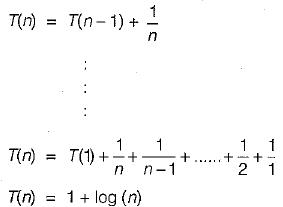⇒ O(log n)

QUESTION: 3

### The concept of order (Big O) is important because

Solution:

Big O notation gives worst case limit for a given problem: Also find out the least upper bound of problem.

QUESTION: 4

Consider the following functions: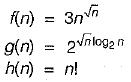Which of the following is true?

Solution: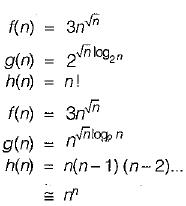Here f(n) is O(g(n))
f(n) is O(h(n))
If f(n) is O(g(n))
f(n) is O(g(n)) and g(n) is O (f(n))

QUESTION: 5

f(n) = 3n2 + 4n + 2
Which will be the exact value for f(n)?

Solution:

f(n) = 3 n2 + 4n + 2
So, f(n) = n2 + n2 + n2 = O (n2)

QUESTION: 6

Which of the following is the average number of key comparisons done by sequential reach in the successful case?

Solution:

in linear or sequential search the maximum number of comparisons are (n) for V elements hence the number of comparisons when the element to be found is at the middle of the array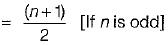= n/2 [If n is even]
In general average case =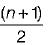QUESTION: 7

Which sort will operate in quadratic time relative to the number of elements in the array (on the average)?

Solution:

Bubbles sort have time complexity of O(n2) and all other have times complexity 0(nlogn).

QUESTION: 8

If one uses straight two-way merge sort algorithm to sort the following elements in ascending order:
20,47,15,8,9,4,40,30,12,17
Then the order of these elements after the 2nd pass of the algorithm is

Solution: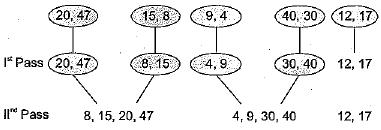QUESTION: 9

The recurrence relation:
T(1) = 2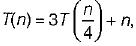, has the solution T(n) equal to

Solution:

Let, 4k = n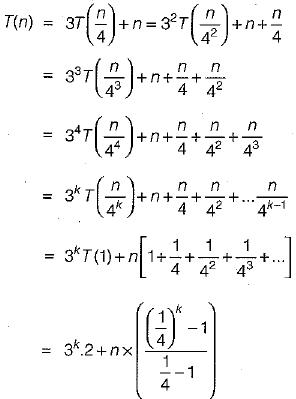≌ O(n)

QUESTION: 10

Consider the following two functions: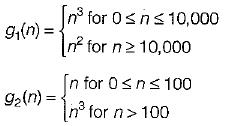Which of the following is true?

Solution: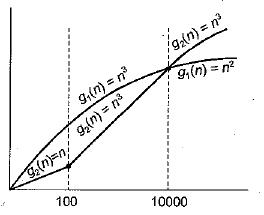Therefore;
n2 ≤ n3 for N ≥ 10000
g1(n) = O(g2(n))